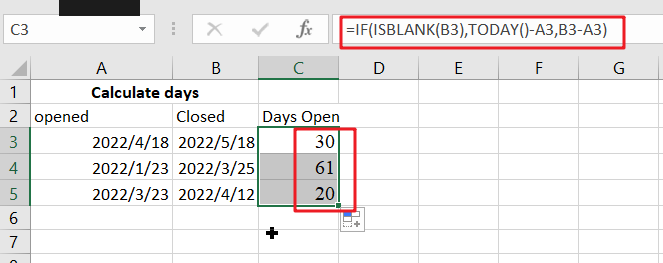# Calculate Days Open in Excel

If you want to know how to Calculate days in Excel, there are some formulas that you can use to do so. For example, you can use the DAYS function in Excel to find the number of days between two dates. However, if you want to find the number of days a ticket has been opened, you can use the if function.

This function calculates the number of days between two dates. It requires the date to be in an Excel-compatible format. Using the IF function, you can also specify the year, month, and day.

## Calculate the Number of Days a Ticket Has Been Opened in Excel

It is important to track the time it takes to resolve support tickets whenever you are dealing with them. You can use Excel’s built-in function to calculate the backlog to do this. When your team members work long hours, the number of tickets they must resolve each day can increase significantly. In order to calculate the backlog, you first need to know the average resolution time of tickets. Once you have the average resolution time, you can subtract the open date from the current date to calculate the days a ticket has been open.To calculate how many days a ticket has been open, you must first determine the date when the ticket was authored. You can also find out the number of days between a ticket’s date of issuance and its closing date by adding up the days between the two dates. Here’s an example. The ticket was created on April 18, 2022, and is still open. You can also calculate the number of days the ticket has been open using Excel. The formula is easy to use and makes analyzing your data easier.

### Generic Formula

`=IF(ISBLANK(closed),TODAY()-opened,closed-opened)`

### Summary:

`=IF(ISBLANK(D5),TODAY()-C5,D5-C5)`If you want to calculate the number of days the spreadsheet has been open, you can use the IF function. In this case, you will need to input a date in a format that Excel understands.

## Conclusion

You can use the IF function to Calculate the number of days a ticket remains open. You can enter the values in the formula, and a calculation will display the number of days that have passed since it was opened. The result of this calculation will be the number of days between the original ticket creation date and the date it was last reopened. By just putting in the values in the manner mentioned above, you can calculate the days opened!

### Related Functions

• Excel ISBLANK function
The Excel ISBLANK function returns TRUE if the value is blank or null.The syntax of the ISBLANK function is as below:= ISBLANK (value)…
• Excel IF function
The Excel IF function perform a logical test to return one value if the condition is TRUE and return another value if the condition is FALSE. The IF function is a build-in function in Microsoft Excel and it is categorized as a Logical Function.The syntax of the IF function is as below:= IF (condition, [true_value], [false_value])….
• Excel TODAY function
The Excel TODAY function returns the serial number of the current date. So you can get the current system date from the TODAY function. The syntax of the TODAY function is as below:=TODAY()…
• Excel DAYS function
The Excel DAYS function returns the number of days between two dates.The syntax of the DAYS function is as below:= DAYS (end_date,start_date)…
Related Posts

If Value is Greater Than A Certain Value

IF function is frequently used in Excel worksheet to return you expect “true value” or “false value” based on the logical test result. If you want to see if a value in one cell is greater than a specific value, ...

If Cell is Not Blank

IF function is frequently used in Excel worksheet to return you expect “true value” or “false value” based on the result of created logical test. If you want to see if a cell is blank or not, and leave some ...

If Cell is Blank

IF function is frequently used in Excel worksheet to return you expect “true value” or “false value” based on the result of created logical test. If you want to see if a cell is blank or not, and leave some ...

If Cell Equals Certain Text String

IF function is frequently used in Excel worksheet to return you expect “true value” or “false value” based on the result of created logical test. If you want to see if cell equals a certain text string like “Win”, you ...

If Cell Contains Either Text1 or Text2

IF function is frequently used in Excel worksheet to return “true value” or “false value” based on the logical test result. If you want to see if cell contains certain substring1 like “abc” or substring2 like “def”, and returns true ...

Sidebar